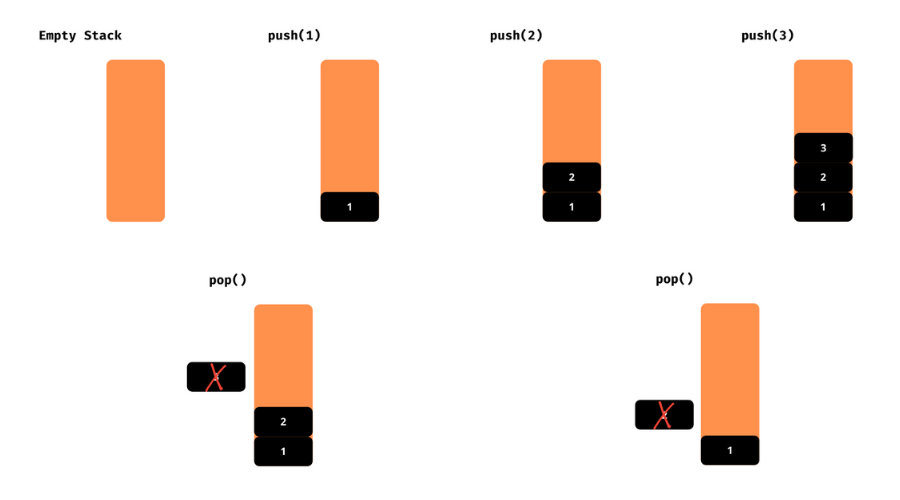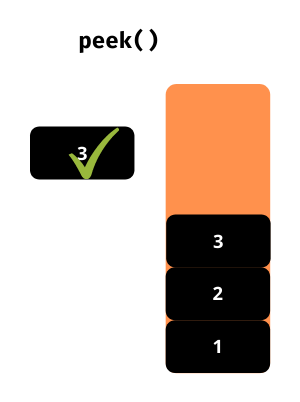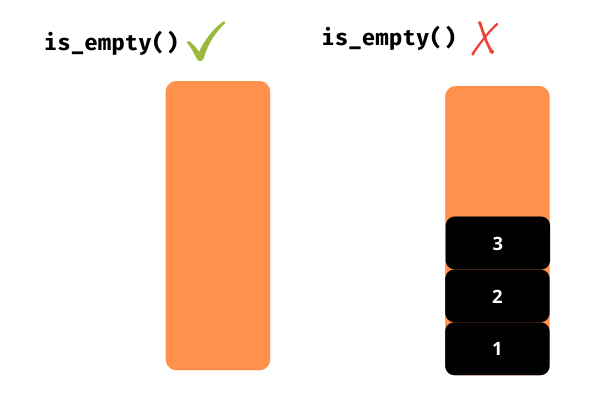• Data structures play a key role in the programming world. They help us to organize our data in a way that can be used efficiently. The stack is one of the simplest data structures.

Let’s learn about the stack and its implementation in Python.

## What is a Stack?

A stack is similar to the pile of books, chairs, etc.., in real-life. And it follows the Last-in/First-out (LIFO) principle. It is the simplest data structure. Let’s see the scenario with a real-world example.

Let’s say we have a pile of books as follows.When we want the third book from the top then, we have to remove the first two books from the top to take out the third book. Here, the topmost book goes last to the pile and comes first of the pile. The data structure stack follows the same principle Last-in/First-out (LIFO) in programming.

## Operations in Stack

There are mainly two operations in a stack

### 1. push(data)

Adds or pushes the data into the stack.

### 2. pop()

Removes or pops the topmost element from the stack.

See the below illustrations of push and pop operations.Let’s see them.

### peek()

Returns the topmost element from the stack.### is_empty()

Returns whether the stack is empty or not.Enough conceptual aspects of the stack data structure. Let’s jump into the implementation without further ado.

I assume you have python installed on your PC if not you can also try the online compiler.

## Stack Implementation

Implementing stack is the easiest one compared to other data structure implementations. We can implement a stack in multiple ways in Python.

Let’s see all of them one by one.

### #1. List

We are going to implement the stack using the list in a class. Let’s see the step by step implementation of the stack.

Step1: Write a class called Stack.

``````class Stack:
pass``````

Step2: We have to maintain the data in a list. Let’s add an empty list in the Stack class with name elements.

``````class Stack:
def __init__(self):
self.elements = []``````

Step3: To push the elements into the stack, we need a method. Let’s write a push method that takes data as an argument and append it to the elements list.

``````class Stack:
def __init__(self):
self.elements = []

def push(self, data):
self.elements.append(data)
return data``````

Step4: Similarly, let’s write the pop method that pops out the topmost element from the stack. We can use the pop method of the list data type.

``````class Stack:
## ...
def pop(self):
return self.elements.pop()``````

We have completed the stack implementation with the required operations. Now, let’s add the helper functions to get more info about the stack.

Step5: We can get the topmost element from the stack using the negative index. The code `element[-1]` returns the last of the list. It is the topmost element of the stack in our case.

``````class Stack:
## ...

def peek(self):
return self.elements[-1]``````

Step6: If the length of the `elements` list is 0, then the stack is empty. Let’s write a method that returns whether the element is empty or not.

``````class Stack:
## ...
def is_empty(self):
return len(self.elements) == 0``````

We have completed implementing the stack using the list data type.

Oh! wait we just implemented it. But, didn’t see how to use it. How to use it then?

Come let’s see how to implement it. We have to create an object for the Stack class to use it. It’s not a big deal. Let’s do it first.

``````class Stack:
## ...

if __name__ == '__main__':
stack = Stack()``````

We have created the stack object and ready to use it. Let’s follow the below steps to test stack operations.

• Check whether the stack is empty or not using the is_empty method. It should return True.
• Push the numbers 1, 2, 3, 4, 5 into the stack using the push method.
• The is_empty method should return False. Check it.
• Print the topmost element using the peek method.
• Pop the element from the stack using the pop method.
• Check the peek element. It should return the element 4.
• Now, pop all the elements from the stack.
• The is_empty method should return True. Check it.

Our stack implementation is completed if it passes all the above steps. Try to write the code for the above steps.

Did you write the code? No, don’t worry check the code below.

``````class Stack:
def __init__(self):
self.elements = []

def push(self, data):
self.elements.append(data)
return data

def pop(self):
return self.elements.pop()

def peek(self):
return self.elements[-1]

def is_empty(self):
return len(self.elements) == 0

if __name__ == '__main__':
stack = Stack()

## checking is_empty method -> true
print(stack.is_empty())

## pushing the elements
stack.push(1)
stack.push(2)
stack.push(3)
stack.push(4)
stack.push(5)

## again checking is_empty method -> false
print(stack.is_empty())

## printing the topmost element of the stack -> 5
print(stack.peek())

## popping the topmost element -> 5
stack.pop()

## checking the topmost element using peek method -> 4
print(stack.peek())

## popping all the elements
stack.pop()
stack.pop()
stack.pop()
stack.pop()

## checking the is_empty method for the last time -> true
print(stack.is_empty())
``````

Hurray! we have completed the stack implementation from scratch using the list data type. You will see the output as mentioned below if you run the above code.

``````True
False
5
4
True``````

We can directly use the list data type as a stack. The above implementation of stack helps you understand the stack implementation in other programming languages as well.

You can also check out these list related articles.

Let’s see the built-in deque from the collections built-in module which can act as a stack.

### #2. deque from collections

It is implemented as a double-ended queue. Since it supports the addition and removal of elements from both ends. Hence we can use it as a stack. We can make it follow the LIFO principle of the stack.

It is implemented using other data structures called the doubly-linked list. So the performance of the insertion and deletion of elements are consistent. Accessing elements from the middle linked list took O(n) time. We can use it as a stack as there is no need to access the middle elements from the stack.

Before implementing the stack, let’s see the methods that are used to implement the stack using the queue.

• append(data) – used to push the data to the stack
• pop() – used to remove the topmost element from the stack

There are no alternative methods for peek and is_empty. We can print the whole stack in place of peek method. Next, we can use the len method to check whether the stack is empty or not.

Let’s implement the stack using deque from the collections module.

``````from collections import deque

## creating deque object
stack = deque()

## checking whether stack is empty or not -> true
print(len(stack) == 0)

## pushing the elements
stack.append(1)
stack.append(2)
stack.append(3)
stack.append(4)
stack.append(5)

## again checking whether stack is empty or not -> false
print(len(stack) == 0)

## printing the stack
print(stack)

## popping the topmost element -> 5
stack.pop()

## printing the stack
print(stack)

## popping all the elements
stack.pop()
stack.pop()
stack.pop()
stack.pop()

## checking the whether stack is empty or not for the last time -> true
print(len(stack) == 0)``````

That’s it. We have learned how to implement stack using the deque from the collections built-in module. You will get the output as mentioned below if you execute the above program.

``````True
False
deque([1, 2, 3, 4, 5])
deque([1, 2, 3, 4])
True``````

Till now, we have seen two ways to implement the stack. Are there any other ways to implement a stack? Yeah! Let’s see the final way to implement a stack in this tutorial.

### #3. LifoQueue

The name LifoQueue itself says that it follows the LIFO principle. Hence we can use it as a stack without any doubt. It is from the built-in module queue. The LifoQueue provides some handy methods that are useful in the stack implementation. Let’s see them

• put(data) – adds or pushes the data to the queue
• get() – removes or pops the topmost element from the queue
• empty() – returns whether the stack is empty or not
• qsize() – returns the length of the queue

Let’s implement the stack using LifoQueue from the queue module.

``````from queue import LifoQueue

## creating LifoQueue object
stack = LifoQueue()

## checking whether stack is empty or not -> true
print(stack.empty())

## pushing the elements
stack.put(1)
stack.put(2)
stack.put(3)
stack.put(4)
stack.put(5)

## again checking whether stack is empty or not -> false
print(stack.empty())

## popping all the elements
print(stack.get())
print(stack.get())
print(stack.get())

## checking the stack size
print("Size", stack.qsize())

print(stack.get())
print(stack.get())

## checking the whether stack is empty or not for the last time -> true
print(stack.empty())
``````

You will get the output mentioned below if you execute the above program without changing it.

``````True
False
5
4
3
Size 2
2
1
True``````

## Application of Stack

Now, you have sufficient knowledge about stacks to apply it in programming problems. Let’s see a problem and solve it using a stack.

Given an expression, write a program to check whether the parentheses, braces, curly-braces are balanced correctly or not.

Let’s see some examples.

Input: “[{}]([])”

Output: Balanced

Input: “{[}]([])”

Output: Not Balanced

We can use the stack to solve the above problem. Let’s see the steps to solve the problem.

• Create a stack to store the characters.
• If the length of the expression is odd, then the expression is Not Balanced
• Iterate through the given expression.
• If the current character is the opening bracket from ( or { or [, then push it to stack.
• Else if the current character is a closing bracket ) or } or ], then pop from the stack.
• If the popped character is matching with the starting bracket then continue else brackets are not balanced.
• After the iteration, if the stack is empty then the equation is Balanced else the equation is Not Balanced.

We can make use of the set data type for brackets match checking.

``````## stack
class Stack:
def __init__(self):
self.elements = []

def push(self, data):
self.elements.append(data)
return data

def pop(self):
return self.elements.pop()

def peek(self):
return self.elements[-1]

def is_empty(self):
return len(self.elements) == 0

def balance_check(expression):
## checking the length of the expression
if len(expression) % 2 != 0:
## not balanced if the length is odd
return False

## for checking
opening_brackets = set('([{')
pairs = set([ ('(',')'), ('[',']'), ('{','}') ])

## stack initialization
stack = Stack()

## iterating through the given expression
for bracket in expression:

## checking whether the current bracket is opened or not
if bracket in opening_brackets:
stack.push(bracket)
else:
## popping out the last bracket from the stack
popped_bracket = stack.pop()

## checking whether popped and current bracket pair
if (popped_bracket, bracket) not in pairs:
return False

return stack.is_empty()

if __name__ == '__main__':
if balance_check('[{}]([])'):
print("Balanced")
else:
print("Not Balanced")

if balance_check('{[}]([])'):
print("Balanced")
else:
print("Not Balanced")``````

We can use the stack to solve many more problems. The above problem is one of them. Try to apply the stack concept wherever you think it best suits you.

### Conclusion

Yah! You have completed the tutorial. I hope you enjoyed the tutorial as much as I do while making it. That’s it for the tutorial.

Happy Coding 🙂 👨‍💻